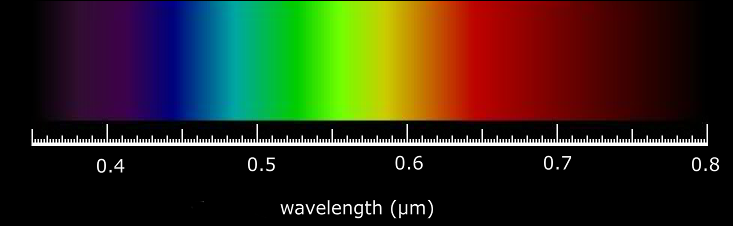# Energy of PhotonA photon is characterized by either a wavelength, denoted by λ or equivalently an energy, denoted by E. There is an inverse relationship between the energy of a photon (E) and the wavelength of the light (λ) given by the equation:

## Photon Energy

where h is Planck's constant and c is the speed of light. The value of these and other commonly used constants are given in the constants page.

h = 6.626 × 10 -34 joule·s

c = 2.998 × 108 m/s

By multiplying to get a single expression, hc = 1.99 × 10-25 joules-m

The above inverse relationship means that light consisting of high energy photons (such as "blue" light) has a short wavelength. Light consisting of low energy photons (such as "red" light) has a long wavelength.

When dealing with "particles" such as photons or electrons, a commonly used unit of energy is the electron-volt (eV) rather than the joule (J). An electron volt is the energy required to raise an electron through 1 volt, thus a photon with an energy of 1 eV = 1.602 × 10-19 J.

Therefore, we can rewrite the above constant for hc in terms of eV:

hc = (1.99 × 10-25 joules-m) × (1ev/1.602 × 10-19 joules) = 1.24 × 10-6  eV-m

Further, we need to have the units be in µm (the units for λ):

hc = (1.24 × 10-6 eV-m) × (106 µm/ m) = 1.24 eV-µm

By expressing the equation for photon energy in terms of eV and µm we arrive at a commonly used expression which relates the energy and wavelength of a photon, as shown in the following equation:

## Photon Energy : Electron-Volt

The exact value of 1 × 106(hc/q) is 1.2398 but the approximation 1.24 is sufficient for most purposes.

To find the energy of a photon at a particular wavelength, click on the map above.